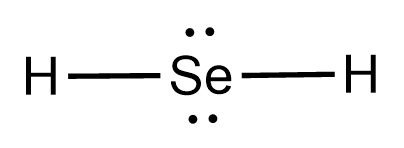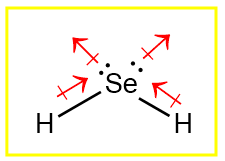# H2Se Lewis Structure, Geometry, Hybridization, and PolaritySelane (H2Se) is a hydride of selenium. It is a covalent compound with a molar mass of 80.987 g/mol. It is a colorless and hazardous gas with a characteristic odor.

It is a selenium analogue of water.

In this article, we will understand the concepts required to predict the lewis structure, geometry, hybridization, and polarity of a given compound.

Contents

## Lewis Structure

There are many methods for representing the structure of a molecule. Gilbert N. Lewis introduced Lewis structures.

In Lewis structures, valence shell electrons are represented by dots around the symbol of chemical elements, and a chemical bond can replace two electrons between atoms.

These structures give an idea of how the electrons are distributed between atoms. It is a 2D representation of a molecule.

There can be more than one lewis structure possible for a molecule. The most stable Lewis structure is the one that satisfies the octet rule and formal charges.

## Octet rule

Atoms form bonds by either losing or gaining or sharing electrons. In the main group, all atoms prefer to have a configuration similar to noble gases as they are most inert and stable. Noble gases have 8 electrons in their valence shell, except He.

Thus, all other atoms lose or gain or share electrons to complete their octet. This preference for electrons is called the octet rule.

For instance, in NaCl, Na has a configuration as 1s2 2s2 2p6 3s1, and to have 8 electrons in the valence shell, one electron from 3s is lost and gained by the 3p of chlorine which has a configuration of 1s2 2s2 2p6 3s2 3p6. The bond formation results in the completion of an octet for both.

## Formal Charge

It is a theoretical concept. It compares the electrons present on an isolated neutral atom with the electrons present on the atom in the compound. It is calculated using the formula

Formal charge= (number of electrons in an isolated atom)-(number of nonbonded electrons)- 0.5*(number of electrons involved in bond formation)

The formal charge is negative when the electrons on the atom in the compound are more than in an isolated atom and positive when the electrons on the atom in the compound are less than in an isolated atom.

When opposite charges are present on adjacent atoms, a bond can replace the charges.

A negative formal charge is more stable on an electronegative atom than on an electropositive atom and vice-versa.

## Steps to Draw Lewis Structure of H2Se

1. Count the total number of valence shell electrons on the compound

Before drawing the structure, we need to know the number of valence shell electrons on all constituent atoms and their sum.

Atom Atomic Number Group Number Valence electrons according to group number Electronic configuration (E.C.) Valence shell from E.C. Valence electrons from E.C.
One Se 34 16 6 1s2 2s2 2p6 3s2 3p6 3d10 4s2 4p4 n=4 6
Two H 1 1 1 1s1 n=1 1
Total number of valence shell electrons= 6 + (2*1) = 8

2. Draw the lewis dot structure for elements.

We draw the Lewis structure of elements by arranging the valence shell electrons around the element’s chemical symbol. The chemical symbols for selenium and hydrogen are Se and H, respectively.

The Lewis dot structure for Se and H are as follows3. Choose a suitable central atom for the compound.

The central atom is supposed to be the least electronegative one out of the constituent atoms as the central atom is supposed to share its electron density with all other atoms.

In H2Se, hydrogen is less electronegative but cannot form more than one bond; hence hydrogen can never be a central atom.

Thus, Se is the central atom for this compound.

4. Draw a skeletal diagram.

In this step, we have to arrange the side atoms and central atom suitably.5. Arrange the valence electrons around the elemental symbols.

The total valence shell electrons (calculated in step 1) are placed according to a predicted bond formation.6. Complete the octet of atoms by forming bonds.Each H has one valence electron in the isolated state. They share one electron with Se to have a fully filled valence shell configuration as only two electrons can be accommodated in the valence shell of hydrogen.

Se has six valence electrons in the isolated state. It shares one electron from all H-atoms, and the octet is completed.

7. Calculate the formal charge on all atoms.

The net charge on this compound is zero. Therefore, the sum of formal charge on three atoms should come out to be zero.

Atom Total number of valence electrons in a free atom Total number of non-bonding electrons (Total number of bonding electrons)*0.5 Formal Charge
Se 6 4 4*0.5=2 6-4-2=0
H1 1 0 2*0.5=1 1-0-1=0
H2 1 0 2*0.5=1 1-0-1=0

Thus, the structure drawn in step 6 is the best Lewis structure for H2Se.

For a better understanding, one can go through this video for a better explanation.

Related Topic

KrF2 Lewis Structure

CO32- Lewis Structure

C2H5OH Lewis Structure

H3PO4 Lewis Structure

CH3OCH3 Lewis Structure

## H2Se Geometry

Geometry is the 3D arrangement of atoms in space. Lewis structure cannot predict geometry correctly as it is quite a primitive concept.

We use valence shell electron pair repulsion theory to predict geometry and shapes.

According to VSEPR theory-

• The valence electron pairs repel each other, and this leads to instability.

• To make the arrangement of the electrons stable, the repulsions between them have to be decreased.

• As a result, electrons align themselves so that the repulsion is the least, and the distance between them is maximum.

• The stable arrangement of the valence electron pairs of atoms helps determine the molecular geometry.

Valence shell electrons involved in bonding are known as bonding pairs of electrons (bp), and those valence shell electrons that are not involved in bonding are termed as lone pairs of electrons (lp).

The terms geometry and shape are often confused with each other. We do not consider the effect of lone pairs in geometry, while we consider the distortions due to lone pairs in shape.

### How to Predict Geometry of H2Se Using VSEPR

1. Count the number of valence shell electrons on the central atom and let it be equal to A (arbitrary variable)

In the case of H2Se, the central atom is Se. Se has 6 valence electrons. (Shown in step1 of drawing lewis structure)

A=6

2. Count the number of side atoms and let it be equal to B (arbitrary variable). In H2Se, there are two side atoms (hydrogen) and B=2

3. If the compound is charged, subtract the charge from B for the positively charged compound and add the charge to B for the negatively charged compound. This step can be avoided for neutral compounds.

In H2Se, there is no contribution of charge and B=2 only.

4. Add the contribution of side atoms and charge to the contribution of the central atom, i.e., A+B

For H2Se, A+B=8

5. Divide A+B by 2 to find total electron pairs affecting the shape.

For H2Se, there are 4 electron pairs.

6. Divide the total electron pairs as bonding and non-bonding. The bonding electron pair is equal to the number of side atoms.

For H2Se, there are two side atoms. Thus, there are two bonding pairs of electrons and two non-bonding pairs of electrons.

Using this information, we can predict geometry and shape using the following table. Electron geometry is tetrahedral, and the shape is bent. The geometry and shape are different for compounds with lone pairs.## H2Se Hybridization

In some polyatomic compounds like methane, we need a new concept to explain the bonding. This concept is hybridization.

Hybridization is the mixing of atomic orbitals, which are similar in energy and size, to form equivalent orbitals. It involves the redistribution of energy.

There is no actual mixing; just mixing of wavefunctions takes place.

For instance, one 3s and three 3p orbitals can mix to form four sp3 hybrid orbitals, but 1s and 5p cannot.

Hybridization has successfully explained bonding in many compounds, but many other compounds do not undergo hybridization.

Drago’s rule tells us the conditions under which a compound does not undergo hybridization. Those conditions are-

• Central atom should belong to third and above periods.

-Se belongs to the third period.

• Side atoms should be less electronegative than the central atom.

-H is less electronegative than Se.

• The central atom has at least one lone pair of electrons.

-Se has two lone pairs.

H2Se satisfies the conditions under which hybridization should not be considered and does not undergo hybridization. Under these conditions, the difference in the energy of atomic orbitals to be mixed is large and hence not suitable.

## H2Se Polarity

The polarity of a compound can be determined by the presence or absence of a net dipole moment. The net dipole moment of a compound depends on-

The dipole moment of the bond

• The difference in electronegativity of atoms constituting the bonds

• Geometry/ symmetry of compound

Dipole moment is a vector quantity. In H2Se, there is only one type of bond, “H-Se.” The electronegativity of Se and H are 2.55 and 2.2, respectively. The difference comes out to be 0.35, which is very small.

Hence, we can say that the bonds are slightly polar and the bond dipole moment is non-zero. The shape of the compound is bent, and dipole moments do not get canceled out. Hence, the compound is polar.

It would have been non-polar if lone pairs were absent on the central atom.## Conclusion

H2Se is an inorganic compound.

The lewis structure drawn in the above sections follows the octet rule and formal charges. The geometry is tetrahedral, and the shape is bent.

H2Se does not undergo hybridization.

It is a polar compound.